# Tamil Nadu Board Class 11 Physics Practical

Practicals form an important part of the Class 11 Physics. Experiment is the basic foundation for the development of science, as well. It helps students to understand the subject or concept thoroughly by giving them hands-on practical experience. Students can now find out about the Tamil Nadu Board Class 11 Physics Practical experiments they need to focus on to get good marks in the exams. Students can refer to the experiments and activities from the TN Board Class 11 Physics Practicals mentioned below and prepare most efficiently for the final exams.

Students can read these practical notes to prepare more efficiently for the examination.

## Tamil Nadu Board Class 11 Physics Practical – List of Experiments

This description given below will inform students about the various lab facilities, measuring instruments, chemicals, glassware, other equipment and such that are made available in the school laboratory.

Description of the experiment in the TN Class 9 Science Practical book follows the given format:

1. Aim of the experiments
2. Apparatus or materials required
3. Formula or principle behind the experiment
4. Diagram
5. Procedure
6. Observations
7. Calculation
8. Result
 1. Moment of Inertia of solid sphere of known mass using Vernier caliper 2. Non-uniform bending – verification of relation between the load and the depression using pin and microscope 3. Spring constant of a spring 4. Acceleration due to gravity using simple pendulum 5. Velocity of sound in air using resonance column 6. Viscosity of a liquid by Stoke’s method 7. Surface tension by capillary rise method 8. Verification of Newton’s law of cooling using calorimeter 9. Study of the relation between the frequency and length of a given wire under constant tension using sonometer 10. Study of the relation between the length of a given wire and tension for constant frequency using sonometer 11. Verification of parallelogram law of forces (Demonstration only- not for examination) 12. Determination of density of a material of wire using screw gauge and physical balance (Demonstration only- Not for examination).

Note: Students should be instructed to perform the experiments given in ICT corner at the end

of each unit of Volume 1. (Self study only- Not for examination)

1. MOMENT OF INERTIA OF A SOLID SPHERE OF KNOWN MASS USING VERNIER CALIPER

AIM: To determine the moment of inertia of a solid sphere of known mass using Vernier caliper

APPARATUS REQUIRED: Vernier caliper, Solid sphere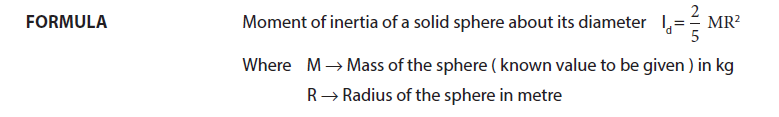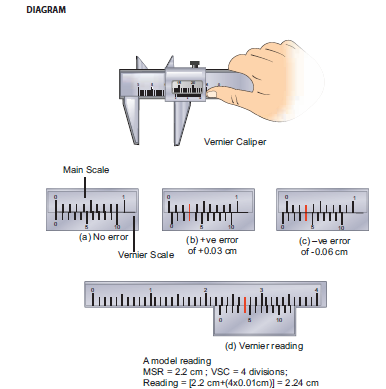PROCEDURE

➩ The Vernier caliper is checked for zero errors and error if found, is to be noted

➩ The sphere is kept in between the jaws of the Vernier caliper and the main scale reading

(MSR) is noted.

➩ Vernier scale division which coincides with some main scale division (VSD ) is noted. Zero

➩ Multiply this VSR by Least Count (LC) and add it with MSR. This will be the diameter of the

sphere.

➩ Observations are to be recorded for different positions of the sphere and the average value

of the diameter is found. From this value radius of the sphere R is calculated.

➩ Using the known value of the mass of the sphere M and calculated radius of the sphere R, the

moment of inertia of the given sphere about its diameter can be calculated using the given

formula.

LEAST COUNT (LC)

One main scale division (MSD) = . . . . . . . . . . . . . . . cm

Number of Vernier scale divisions = . . . . . .OBSERVATIONS

Zero error =

Zero correction =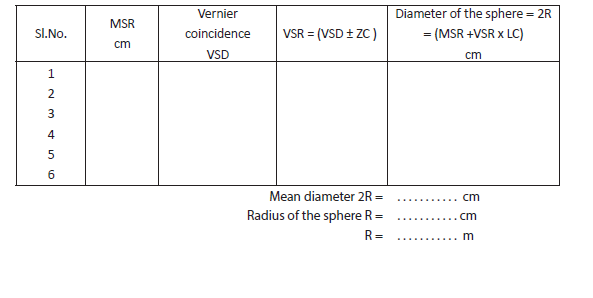CALCULATION:

Mass of the sphere M = . . . . . . . . . . . . . kg

(Known value is given )

Radius of the sphere R =. . . . . . . . . . . . .metre

Moment of inertia of a solid sphere about its diameter Id = ⅖ MR2 = kg m2

Result:

The moment of inertia of the given solid sphere about its diameter using

Vernier caliper Id = ………………….. kg m2

2. NON – UNIFORM BENDING – VERIFICATION OF RELATION BETWEEN

LOAD AND DEPRESSION USING PIN AND MICROSCOPE

AIM: To verify the relation between the load and depression using non-uniform bending of a beam.

APPARATUS REQUIRED: A long uniform beam (usually a metre scale), two knife – edge

supports, mass hanger, slotted masses, pin, vernier microscope.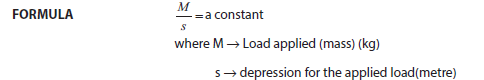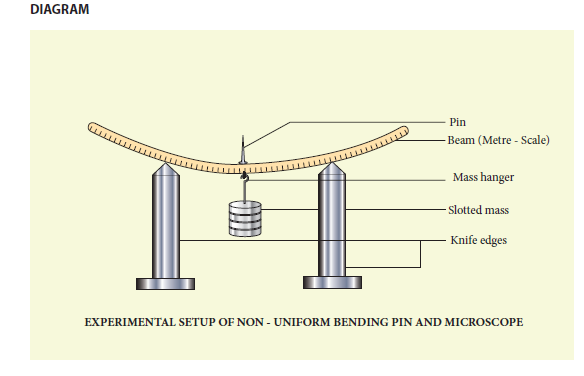PROCEDURE

➩ Place the two knife – edges on the table.

➩ Place the uniform beam (metre scale) on top of the knife edges.

➩ Suspend the mass hanger at the centre. A pin is attached at the centre of the scale where the

hanger is hung.

➩ Place a vernier microscope in front of this arrangement

➩ Adjust the microscope to get a clear view of the pin

➩ Make the horizontal cross-wire on the microscope to coincide with the tip of the pin. (Here

➩ Note the vertical scale reading of the vernier microscope

➩ Add the slotted masses one by one in steps of 0.05 kg (50 g) and take down the readings.

depressions for the corresponding load M.

OBSERVATIONS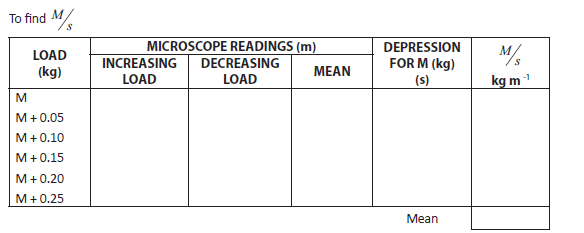MODEL GRAPH

A graph between M and s can be drawn by taking M along X- axis and s along Y – axis.

This is a straight line.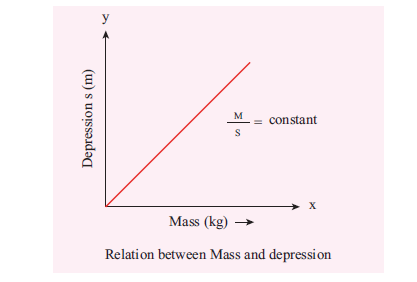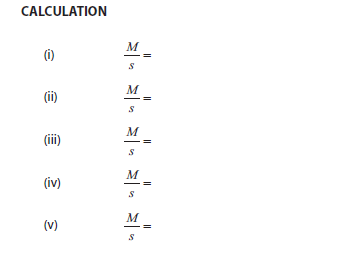RESULT

➩ The ratio between mass and depression for each load is calculated. This is found to be

Constant.

➩ Thus the relation between load and depression is verified by the method of non-uniform

bending of a beam.

3. SPRING CONSTANT OF A SPRING

AIM: To determine the spring constant of a spring by using the method of vertical oscillations

APPARATUS REQUIRED: Spring, rigid support, hook, 50 g mass hanger, 50 g slotted masses, stop clock, metre scale, pointer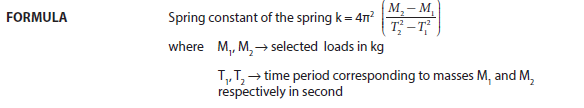Diagram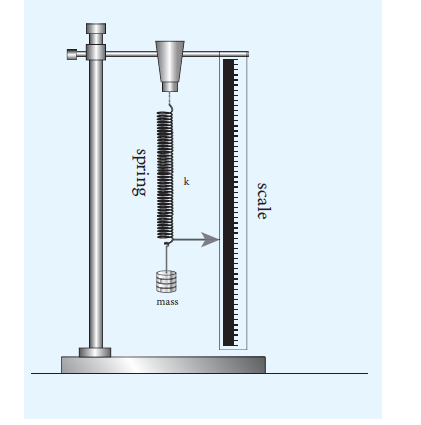Procedure

➩ A spring is firmly suspended vertically from a rigid clamp of a wooden stand at its upper end

with a mass hanger attached to its lower end. A pointer fixed at the lower end of the spring

moves over a vertical scale fixed.

➩ A suitable load M (eg; 100 g ) is added to the mass hanger and the reading on the scale at

which the pointer comes to rest is noted. This is the equilibrium position.

➩ The mass in the hanger is pulled downward and released so that the spring oscillates

vertically on either side of the equilibrium position.

➩ When the pointer crosses the equilibrium position a stop clock is started and the time taken

for 10 vertical oscillations is noted. Then the period of oscillation T is calculated.

➩ The experiment is repeated by adding masses in steps of 50 g to the mass hanger and period

of oscillation at each time is calculated.

➩ For the masses M1 and M2 ( with a difference of 50 g ), if T1 and T2 are the corresponding

periods, then the value M2 – M, / T2 2 – T12 is calculated and its average is found.

➩ Using the given formula the spring constant of the given spring is calculated.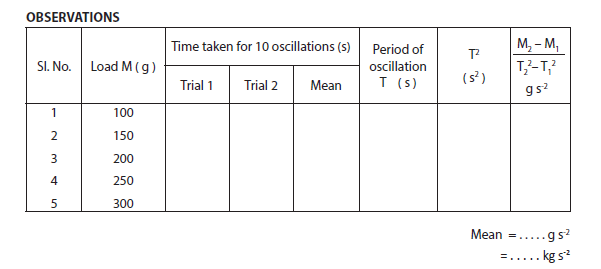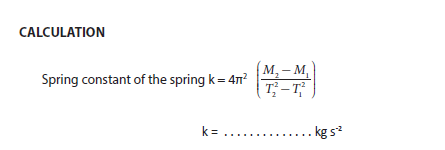RESULT

The spring constant of the given spring k = . . . . . . . . . . . . kg s-2

4 ACCELERATION DUE TO GRAVITY USING SIMPLE PENDULUM

AIM : To measure the acceleration due to gravity using a simple pendulum

APPARATUS REQUIRED: Retort stand, pendulum bob, thread, meter scale, stop watch.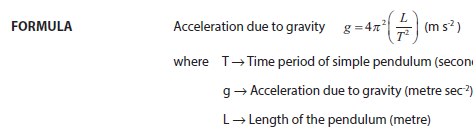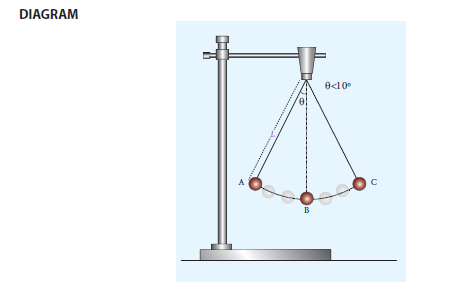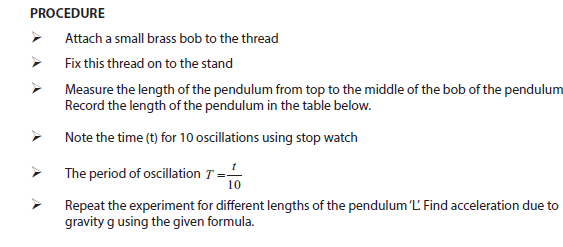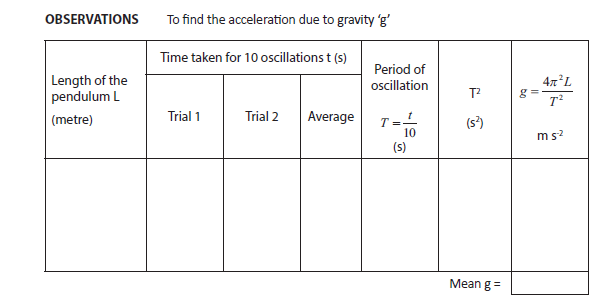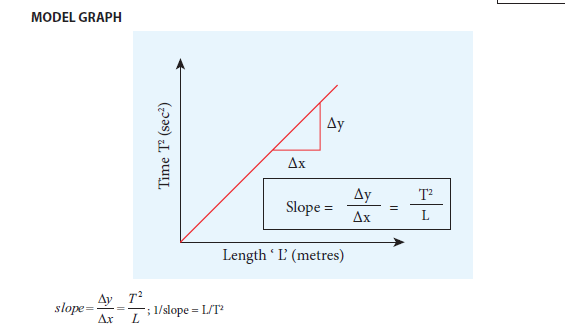RESULT

The acceleration due to gravity ‘g’ determined using simple pendulum is

i) By calculation = . . . . . . . . . m s-2

ii) By graph = . . . . . . . . . m s-2

5. VELOCITY OF SOUND IN AIR USING RESONANCE COLUMN

AIM: To determine the velocity of sound in air at room temperature using the resonance column.

APPARATUS REQUIRED: Resonance tube, three tuning forks of known frequencies, a rubber hammer, one thermometer, plumb line, set squares, water in a beaker.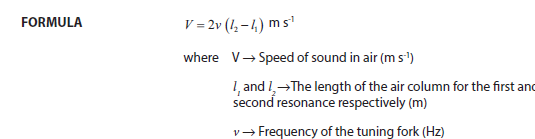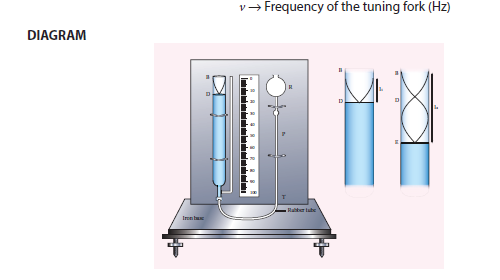PROCEDURE

➩ The inner tube of the resonance column is lowered so that the length of air column inside

the tube is very small.

➩ Take a tuning fork of known frequency and strike it with a rubber hammer. The tuning fork

now produces longitudinal waves with a frequency equal to the natural frequency of the

tuning fork.

➩ Place the vibrating tuning fork horizontally above the tube. Sound waves pass down the

total tube and reflect back at the water surface.

➩ Now, raise the tube and the tuning fork until a maximum sound is heard.

➩ Measure the length of air column at this position. This is taken as the first resonating

length, l1

➩ Then raise the tube approximately about two times the first resonating length. Excite the

tuning fork again and place it on the mouth of the tube.

➩ Change the height of the tube until the maximum sound is heard.

➩ Measure the length of air column at this position. This is taken as the second resonating

length l2

➩ We can now calculate the velocity of sound in air at room temperature by using the relation.

V = 2v(l2 − l1)

➩ Repeat the experiment with forks of different frequency and calculate the velocity.

➩ The mean of the calculated values will give the velocity of sound in air at room temperature.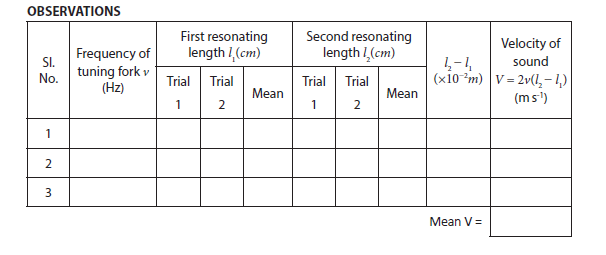CALCULATION

Room temperature, t = ____________ ° C

Velocity of sound in air at room temperature, V = 2v(l2 − l1) = ____________ m s-1

RESULT

Velocity of sound in air at room temperature, (V) = ____________ m s-1

&nbsp;

6. VISCOSITY OF A LIQUID BY STOKE’S METHOD

AIM: To determine the coefficient of viscosity of the given liquid by stoke’s method

APPARATUS REQUIRED: A long cylindrical glass jar, highly viscous liquid, metre scale,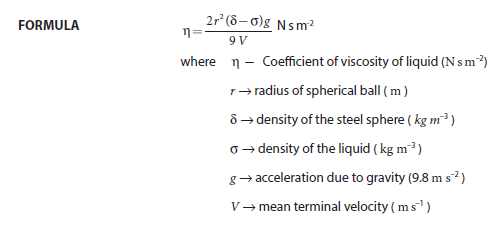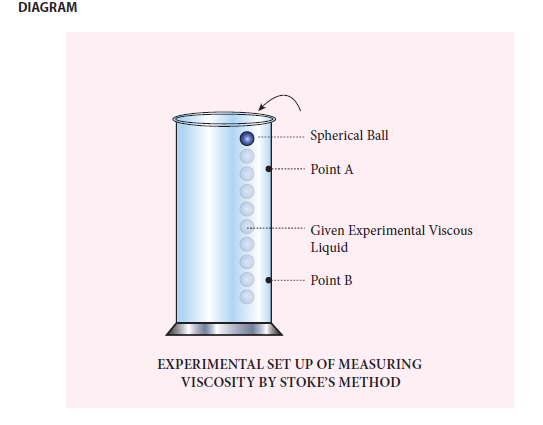PROCEDURE

➩ A long cylindrical glass jar with markings is taken.

➩ Fill the glass jar with the given experimental liquid.

➩ Two points A and B are marked on the jar. The mark A is made well below the surface of the

liquid so that when the ball reaches A it would have acquired terminal velocity V.

➩ The radius of the metal spherical ball is determined using screw gauge.

➩ The spherical ball is dropped gently into the liquid.

➩ Start the stop clock when the ball crosses the point A. Stop the clock when the ball

reaches B.

➩ Note the distance between A and B and use it to calculate terminal velocity.

➩ Now repeat the experiment for different distances between A and B. Make sure that the

point A is below the terminal stage.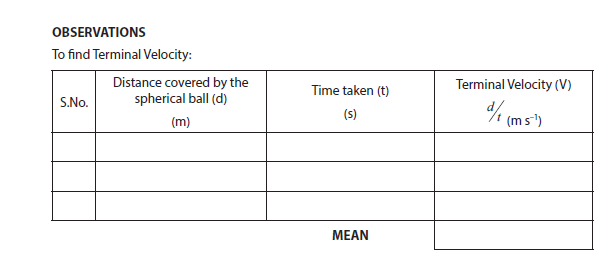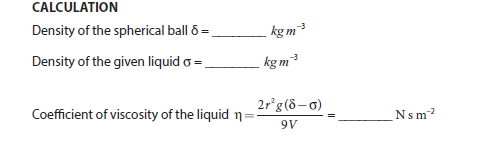RESULT

The coefficient of viscosity of the given liquid by stoke’s method η = ________ N s m–2

7. SURFACE TENSION BY CAPILLARY RISE METHOD

AIM: To determine surface tension of a liquid by capillary rise method.

APPARATUS REQUIRED: A beaker of Water, capillary tube, vernier microscope, two holed rubber stopper, a knitting needle, a short rubber tubing and retort clamp.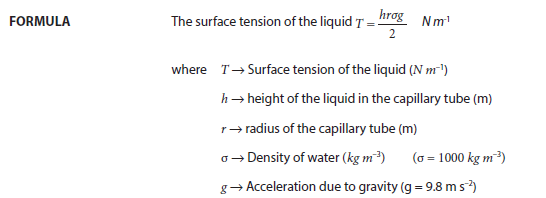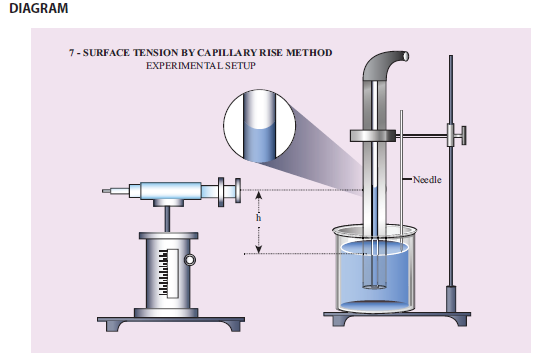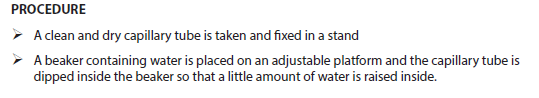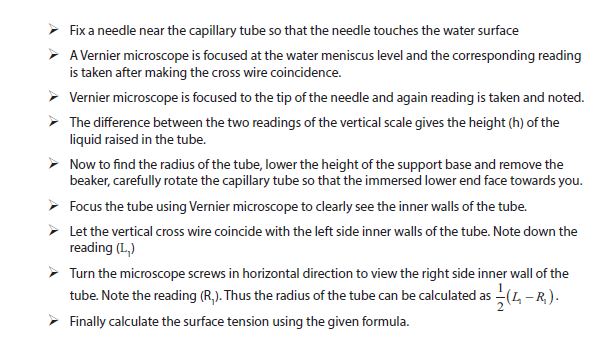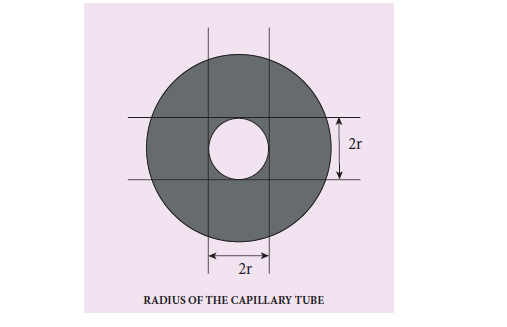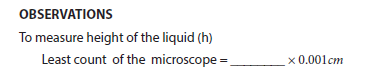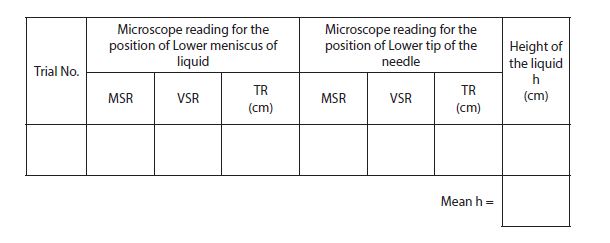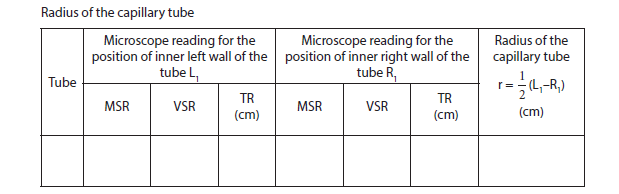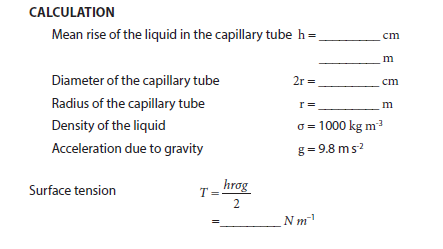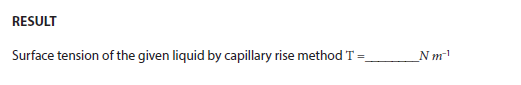8. NEWTON’S LAW OF COOLING USING CALORIMETER

AIM: To study the relationship between the temperature of a hot body and time by plotting a cooling curve.

APPARATUS REQUIRED: Copper calorimeter with stirrer, one holed rubber cork,

thermometer, stop clock, heater / burner, water, clamp and stand

NEWTON’S LAW OF COOLING: Newton’s law of cooling states that the rate of change of the temperature of an object is proportional to the difference between its own temperature and the ambient temperature.

(i.e., the temperature of its surroundings)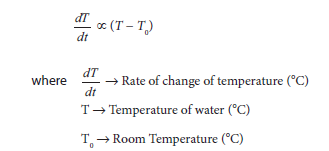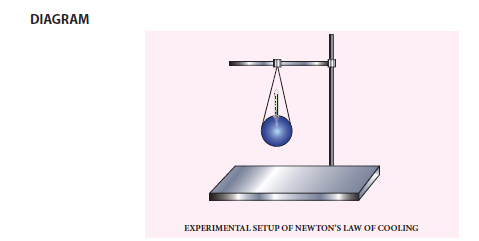PROCEDURE

➩ Note the room temperature as (T0) using the thermometer.

➩ Hot water about 90°C is poured into the calorimeter.

➩ Close the calorimeter with one holed rubber cork

➩ Insert the thermometer into calorimeter through the hole in rubber cork

➩ Start the stop clock and observe the time for every one degree fall of temperature from 80°C

➩ Take sufficient amount of reading, say closer to room temperature

➩ The observations are tabulated

➩ Draw a graph by taking time along the x axis and excess temperature along the y axis.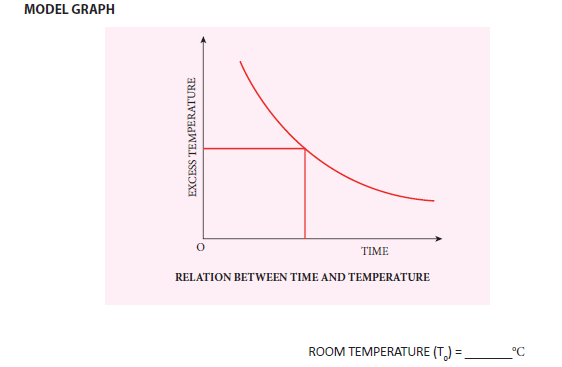OBSERVATIONS

Measuring the change in temperature of water with time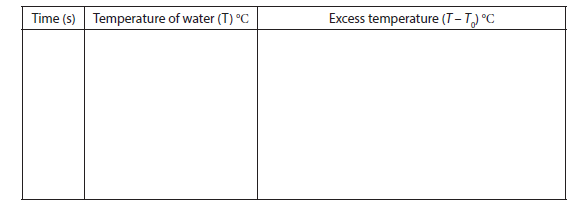RESULT

The cooling curve is plotted and thus Newton’s law of cooling is verified.

9. STUDY OF THE RELATION BETWEEN FREQUENCY AND LENGTH OF A GIVEN WIRE UNDER CONSTANT TENSION USING SONOMETER

AIM: To study the relation between frequency and length of a given wire under constant tension using a sonometer.

APPARATUS REQUIRED: Sonometer, six tuning forks of known frequencies, Metre scale,

rubber pad, paper rider, hanger with half – kilogram masses, wooden bridges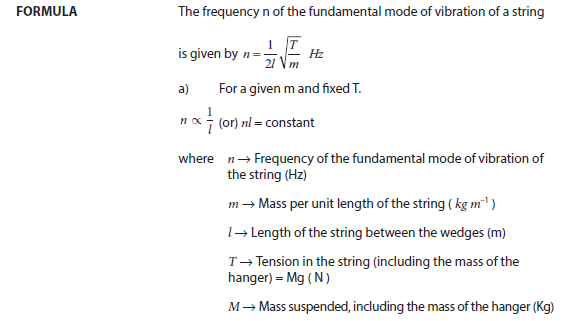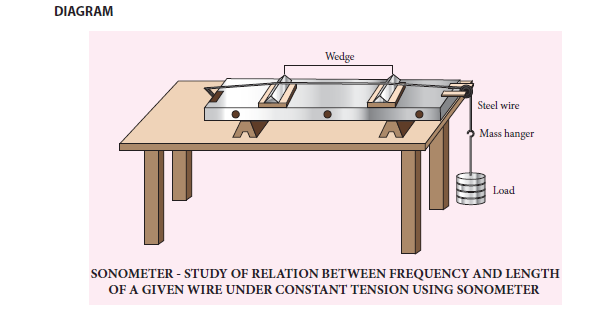PROCEDURE

➩ Set up the sonometer on the table and clean the groove on the pulley to ensure minimum

friction.

➩ Stretch the wire by placing suitable mass in the hanger.

➩ Set the tuning fork into vibrations by striking it against the rubber pad. Plug the sonometer

wire and compare the two sounds.

➩ Adjust the vibrating length of the wire by sliding the bridge B till the sounds appear alike.

➩ For the final adjustment, place a small paper rider R in the middle of the wire AB.

➩ Sound the tuning fork and place its shank stem on the bridge A or on the sonometer box

and slowly adjust the position of bridge B until the paper rider is agitated violently indicating

resonance.

➩ The length of the wire between the wedges A and B is measured using meter scale. It is the

resonant length. Now the frequency of vibration of the fundamental mode equals the frequency

of the tuning fork.

➩ Repeat the above procedure for other tuning forks by keeping the same load in the hanger.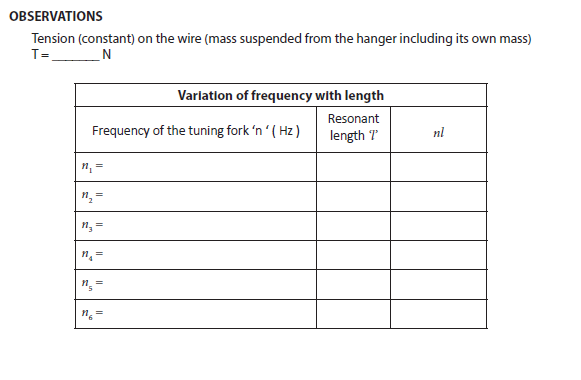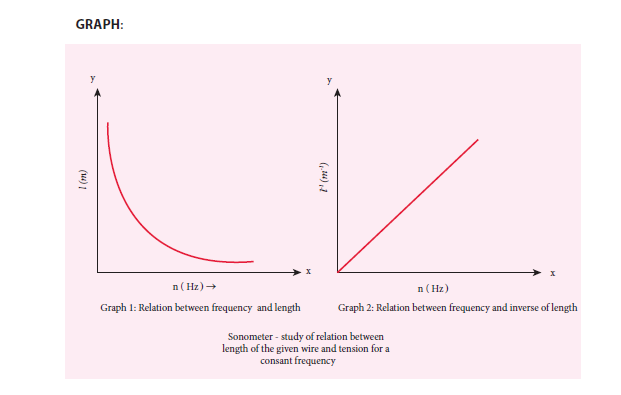CALCULATION

The product nl for all the tuning forks remain constant (last column in the table)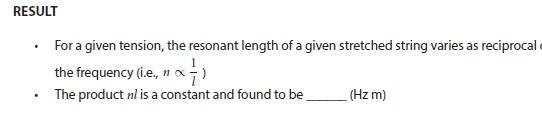10. STUDY OF THE RELATION BETWEEN THE LENGTH OF THE GIVEN WIRE AND TENSION FOR A CONSTANT FREQUENCY USING SONOMETER

AIM: To study the relationship between the length of a given wire and tension for constant frequency using a sonometer

APPARATUS REQUIRED: Sonometer, tuning fork of known frequency, meter scale, rubber

pad, paper rider, hanger with half – kilogram masses, wooden bridges.

FORMULA: The frequency of the fundamental mode of vibration of a string is given by,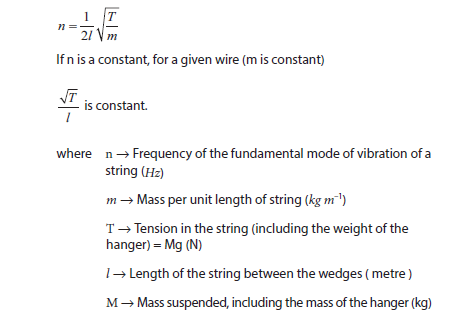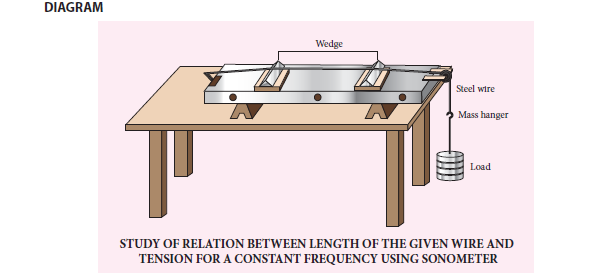PROCEDURE

➩ Set up the sonometer on the table and clean the groove on the pulley to ensure that it has

minimum friction.

➩ Set a tuning fork of known frequency into vibration by striking it against the rubber pad.

Plug the sonometer wire and compare the sound due to the vibration of tuning fork and the

plugged wire.

➩ Adjust the vibrating length of the wire by adjusting the bridge B till the two sounds appear

alike.

➩ Place a mass of 1 kg for initial reading in the load hanger.

➩ For final adjustment place a small paper rider R in the middle of the wire AB.

➩ Now, strike the tuning fork and place its shank stem on the bridge A and then slowly adjust

the position of the bridge B till the paper rider is agitated violently (might eventually falls)

indicating resonance.

➩ Measure the length of the wire between wedges at A and B which is the fundamental mode

corresponding to the frequency of the tuning fork.

➩ Increase the load on the hanger in steps of 0.5 kg and each time find the resonating length

as done before with the same tuning fork.

➩ Record the observations in the tabular column.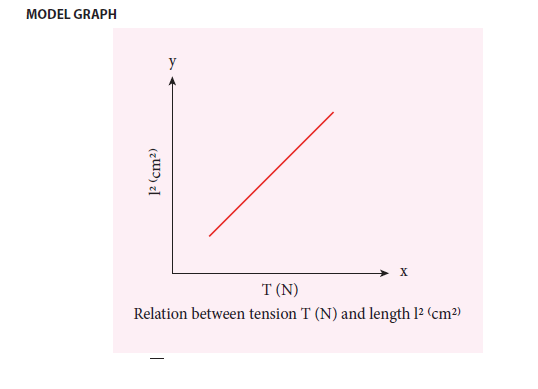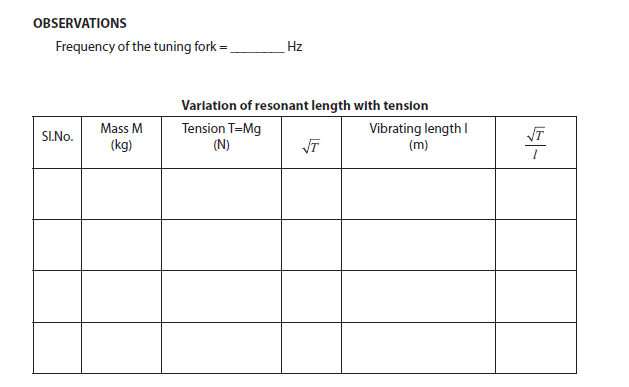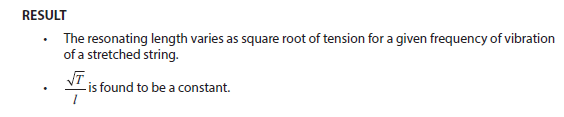Students can access the detailed list of practical experiments and observations from the downloadable PDFs given in the article.